# Kinetic Energy of Rotation

### Kinetic energy of Rotation

• From equation(4) we know that magnitude of velocity of the ith particle in a rigid body rotating about a fixed axis is

vi=ri(dθ/dt) =riω where ri is the distance of particle from the axis of rotation and ω is the velocity of the particle
• Kinetic energy of the ith particle of mass mi is given by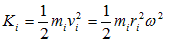• The total kinetic energy of the rigid body as a whole would be equal to the sum of KEs of all particles in the body thus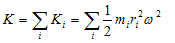• Since angular velocity ω is same for all the particles in the body so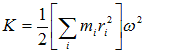• The quantity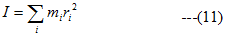tell us how the mass of the rotating body is distributed about the axis of rotation is known as rotational inertia or moment of inertia of the rotating body
• Moment of inertia of the rigid body can be obtained by imaging the body to be subdivided into large number of particles ,the mass of the each particle is then multiplexed by its squared distance from the axis and then summing over these products for all the particles in the body
• Si unit of moment of inertia is Kgm2
• So rotational kinetic energy of a body can now be written as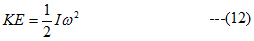• Above expression of rotational kinetic energy KE of a rotating rigid body is analogous to the translational kinetic energy where moment of inertia is analogous to mass m (or inertia) and angular velocity is analogous to velocity v
• Moment of inertia not only depends on the mass but also on how this mass is distributed about the axis of rotation and it must be specified first before calculating moment of inertia of any body
• If the body under consideration is of continuous distribution of matter instead of discrete then moment of inertia is evaluated by means of integration rather then that by summation and this point will be discussed in more detail in our next topic